International
Tables for
Crystallography
Volume D
Physical properties of crystals
Edited by A. Authier

International Tables for Crystallography (2006). Vol. D, ch. 1.1, pp. 15-16

## Section 1.1.4.6.2. The operator A is in diagonal form

A. Authiera*

aInstitut de Minéralogie et de la Physique des Milieux Condensés, Bâtiment 7, 140 rue de Lourmel, 75015 Paris, France
Correspondence e-mail: aauthier@wanadoo.fr

| top | pdf |

#### 1.1.4.6.2.1. Introduction

| top | pdf |

If one takes as the system of axes the eigenvectors of the operator A, the matrix is written in the form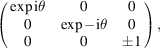where θ is the rotation angle,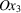is taken parallel to the rotation axis and coefficientis equal to +1 or −1 depending on whether the rotation axis is direct or inverse (proper or improper operator).

The equations (1.1.4.1)can then be simplified and reduce to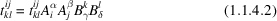(without any summation).

If the product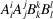(without summation) is equal to unity, equation (1.1.4.2)is trivial and there is significance in the component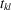. On the contrary, if it is different from 1, the only solution for (1.1.4.2)is that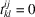. One then finds immediately that certain components of the tensor are zero and that others are unchanged.

#### 1.1.4.6.2.2. Case of a centre of symmetry

| top | pdf |

All the diagonal components are in this case equal to −1. One thus has:

 (i) Tensors of even rank,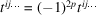. The components are not affected by the presence of the centre of symmetry. The reduction of tensors of even rank is therefore the same in a centred group and in its noncentred subgroups, that is in any of the 11 Laue classes: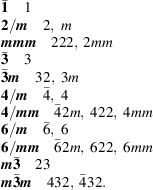If a tensor is invariant with respect to two elements of symmetry, it is invariant with respect to their product. It is then sufficient to make the reduction for the generating elements of the group and (since this concerns a tensor of even rank) for the 11 Laue classes. (ii) Tensors of odd rank,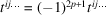. All the components are equal to zero. The physical properties represented by tensors of rank 3, such as piezoelectricity, piezomagnetism, nonlinear optics, for instance, will therefore not be present in a centrosymmetric crystal.

#### 1.1.4.6.2.3. General case

| top | pdf |

By replacing the matrix coefficients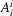by their expression, (1.1.4.2)becomes, for a proper rotation,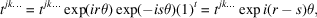where r is the number of indices equal to 1, s is the number of indices equal to 2, t is the number of indices equal to 3 and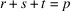is the rank of the tensor. The component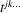is not affected by the symmetry operation if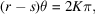where K is an integer, and is equal to zero if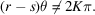The angle of rotation θ can be put into the form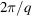, where q is the order of the axis. The condition for the component not to be zero is then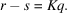The condition is fulfilled differently depending on the rank of the tensor, p, and the order of the axis, q. Indeed, we have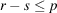and,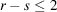: the result of the reduction will be the same for any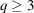;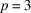,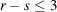: the result of the reduction will be the same for any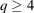;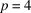,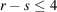: the result of the reduction will be the same for any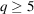.

It follows that:

 (i) for tensors of rank 2, the reduction will be the same for trigonal (threefold axis), tetragonal (fourfold axis) and hexagonal (sixfold axis) groups; (ii) for tensors of rank 3, the reduction will be the same for tetragonal and hexagonal groups; (iii) for tensors of rank 4, the reduction will be different for trigonal, tetragonal and hexagonal groups.

The inconvenience of the diagonalization method is that the vectors and eigenvalues are, in general, complex, so in practice one uses another method. For instance, we may note that equation (1.1.4.1)can be written in the case ofby associating with the tensor amatrix T: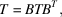where B is the symmetry operation. Through identification of homologous coefficients in matrices T and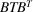, one obtains relations between components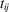that enable the determination of the independent components.